# Solving Exponential Equations With LogarithmsHi there. In this mathematics post, I cover the concept of solving exponential equations with the use of logarithms. This is a technical algebra/calculus topic. It is assumed that the reader is familiar with exponents and logarithm basics.

For the math text I use a combination of QuickLatex.com, witeboard.com and my Wacom stylus and writing tablet.Pixabay Image Source

## Review of Logarithms

Before I get into solving exponential equations with the use of logarithms, I think it is a good idea to do a short review on logarithms.

The logarithm can be viewed as an exponent when it comes to solve exponential questions like

Two to the power of x is equal to 32: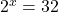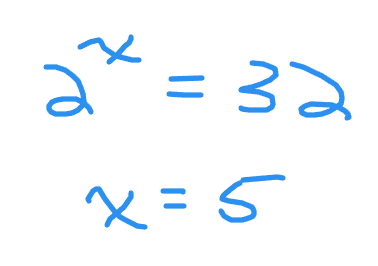For this question the answer is 5 as to the power of 5 is 32.

Logarithm Properties

There are algebra properties with logarithms. I feature three of the common properties.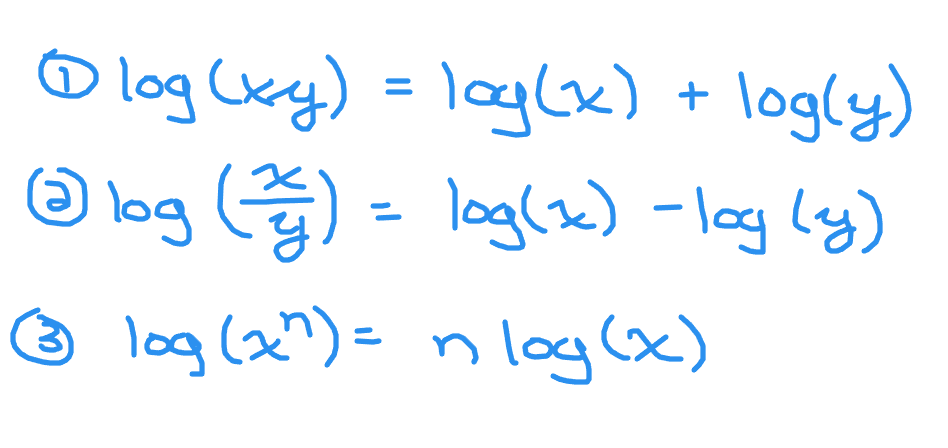The logarithm property that is featured when it comes to solving logarithms is the third one above. That is the exponent in the input of the logarithm can be moved to the front of the logarithm.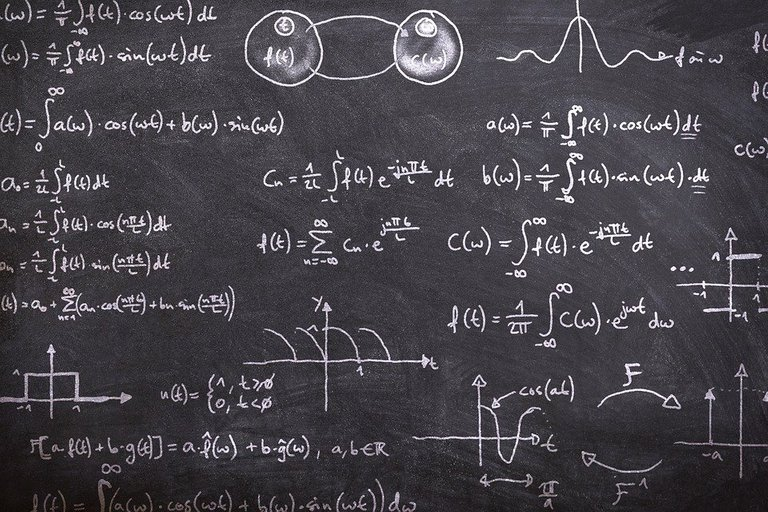Pixabay Image Source

## Examples

Example One

Determine the value of `x` in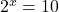.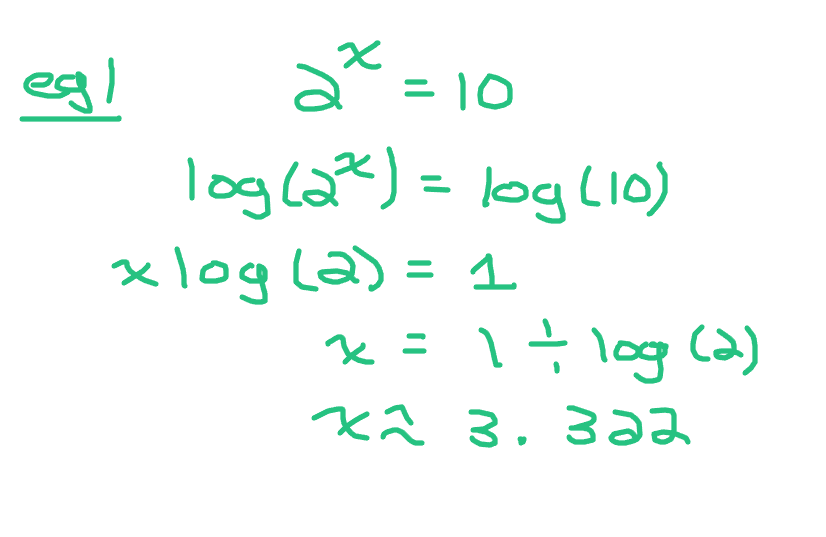For this question logarithms are needed as it is not obvious what the value of `x` is in two to the power of `x` equals ten. Two to the power of 1 is 2, two to the power of two is 4, two with and exponent of 3 is 8 and two to the power of four is 16. The answer is somewhere between 3 and 4.

Take the logarithm of both sides to start. Then solve for `x`. See the screenshot below for details. Note that the logarithm of 10 is one as ten to the power of 1 is 10. Assume base ten for logarithms if there is no subscript.

Example Two

What is `x` in ten to the power of `x` equal to 200? That is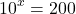.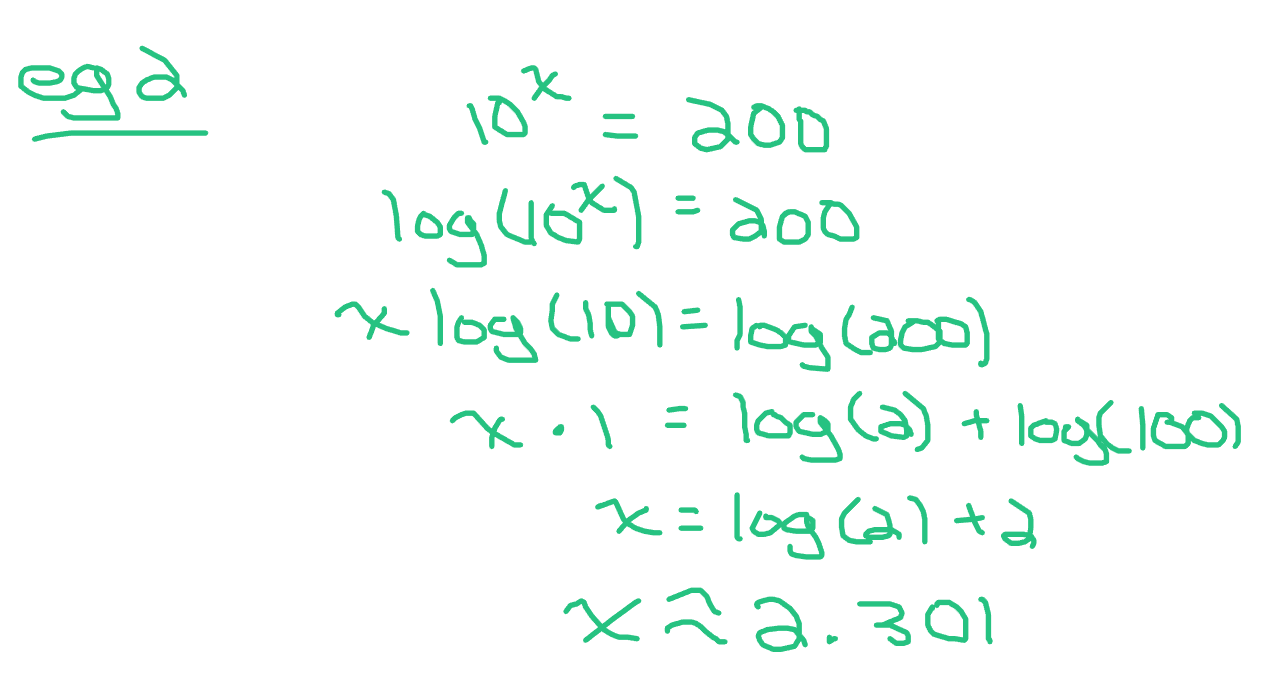To start take the logarithm of ten to the power of `x` on the left side and take the logarithm of 200 on the right side.

Use the power rule property on the left side to move the exponent `x` in front of the logarithm of 10. The logarithm of 10 is 1 if the base is 10.

The answer here is the logarithm of 200 which is about 2.301.

Example Three

What is the value of `x` in one half to the power of `x` equal to 5? That is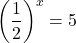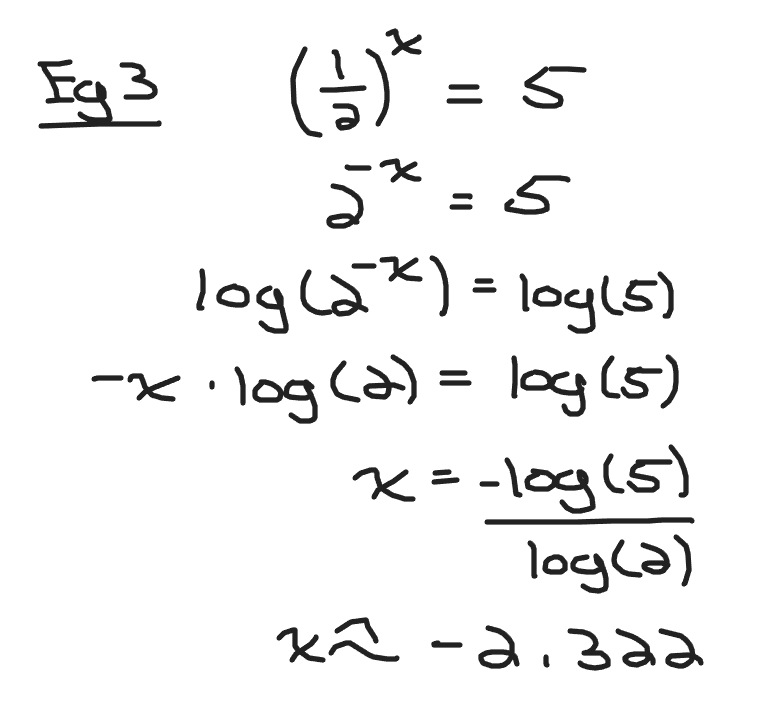Rewrite the one half as two to the power of negative one. The left side becomes two to the power of negative x.

Take the logarithm in both sides. The left side would be the logarithm of two to the power of negative `x`. The right side is the logarithm of five.

From the power rule property for logarithms move the negative `x` to the front of the logarithm.
Divide both sides by the negative logarithm of two. The answer for x is negative logarithm of 5 divided by the logarithm of 2. This is approximately `-2.322`.

Example Four

In seven times three to the power of `2x` equal to 70 what is the value of `x`? That is what is `x` in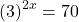?Start with dividing both sides by seven. The left is side is three to the power of `2x` with the right side being 10.

Take the logarithm in both sides. In the left side move the `2x` exponent to the front of the logarithm. On the right side the logarithm of 10 is 1.

Divide both sides by twice the logarithm of 3.

The value of `x` is 1 divided by 2 log 3. This is approximately 1.048.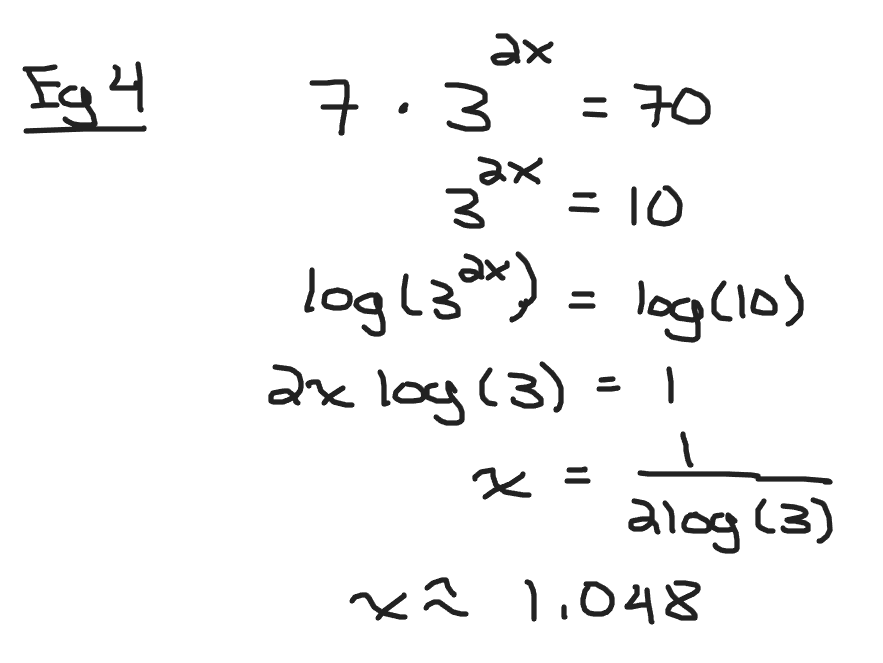Pixabay Image Source

Posted with STEMGeeks

0
0
0.000Logarithms used to seem intimidating back in high school until one of my math teachers clarified with a story from when he was a student.

"Exponent, exponent, iiiisssss? all the kids paused It's an exponent, stupid."

!discovery 37

0
0
0.000This post was shared and voted inside the discord by the curators team of discovery-it
Discovery-it is also a Witness, vote for us here
Delegate to us for passive income. Check our 80% fee-back Program
0
0
0.000Thanks for your contribution to the STEMsocial community. Feel free to join us on discord to get to know the rest of us!

Please consider delegating to the @stemsocial account (85% of the curation rewards are returned).

You may also include @stemsocial as a beneficiary of the rewards of this post to get a stronger support.

0
0
0.000